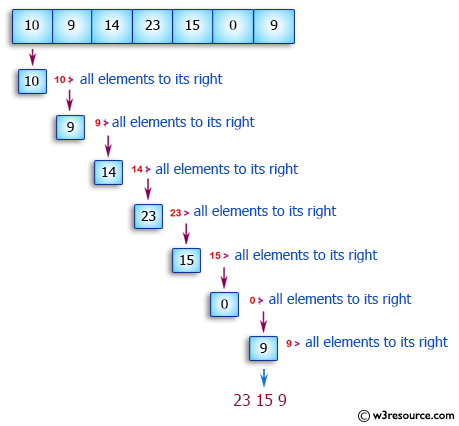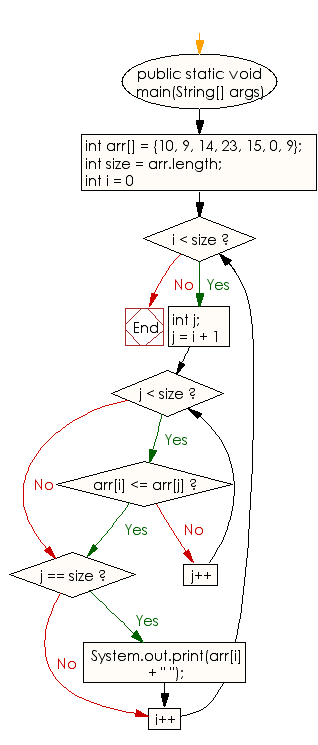﻿ Java exercises: Print all the LEADERS in the array - w3resource# Java Array Exercises: Print all the LEADERS in the array

## Java Array: Exercise-39 with Solution

Write a Java program to print all the LEADERS in the array.

Note: An element is leader if it is greater than all the elements to its right side.

Pictorial Presentation:Sample Solution:

Java Code:

``````import java.util.HashMap;
import java.util.Map;
import java.util.Iterator;
import java.util.Arrays;

public class Main
{
public static void main(String[] args)
{
int arr[] = {10, 9, 14, 23, 15, 0, 9};
int size = arr.length;
for (int i = 0; i < size; i++)
{
int j;
for (j = i + 1; j < size; j++)
{
if (arr[i] <= arr[j])
break;
}
if (j == size)
System.out.print(arr[i] + " ");
}
}
}
```
```

Sample Output:

```
23 15 9
```

Flowchart:Visualize Java code execution (Python Tutor):

Java Code Editor:

Improve this sample solution and post your code through Disqus

What is the difficulty level of this exercise?

Test your Programming skills with w3resource's quiz.

﻿

## Java: Tips of the Day

countOccurrences

Counts the occurrences of a value in an array.

Use Arrays.stream().filter().count() to count total number of values that equals the specified value.

```public static long countOccurrences(int[] numbers, int value) {
return Arrays.stream(numbers)
.filter(number -> number == value)
.count();
}
```

Ref: https://bit.ly/3kCAgLb